# 1 2021-09-14

## 1.1 14:45

Terms:

• Cartan subgroup

• Centralizer of a maximal torus.
• Borel subgroup

• Maximal connected solvable subgroup
• Why care: critical to structure theory of simple reductive algebraic group. Uses pairs $$(B, N)$$ where $$N = N_G(T)$$ is the normalizer of a maximal torus.
• Parabolic subgroup

• Literally any $$P\leq G$$ such that $$B \subseteq P \subseteq G$$
• $$G/P$$ is a complete variety, so all projections $$X\times ({-}) \to ({-})$$ are closed maps.
• $$G/B$$ is the largest complete variety since $$B \subseteq P$$ for all $$P$$.
• Local field

• Complete with respect to a topology induced by $$v$$ a discrete valuation with $$\kappa$$ finite.
• Valuation: $$v: k \to G\cup\left\{{\infty}\right\}$$, $$G\in {\mathsf{Ab}}$$ totally ordered.

• Value group: $$\operatorname{im}v$$
• Valuation ring: $$R_v \mathrel{\vcenter{:}}=\left\{{v(x) \geq 0}\right\}$$
• Prime/maximal ideal: $${\mathfrak{m}}_v \mathrel{\vcenter{:}}=\left\{{v(x)>0}\right\}$$
• Residue field $$\kappa_v \mathrel{\vcenter{:}}= R_v/{\mathfrak{m}}_v$$
• Places: $$\left\{{v}\right\}/\sim$$ where $$v_2\sim v_1 \iff v_2 = \phi \circ v_1$$.
• Uniformizer: for $$R$$ a DVR, a generator $$\pi$$ for the unique maximal ideal, so $$R^{\times}\left\langle{\pi}\right\rangle = R$$ and $$x\in R \implies x = u\pi^k$$
• Global field: algebraic number fields, function fields of algebraic curves over finite fields (so finite extensions of $${\mathbb{F}}_q { \left( {(} \right) } t))$$.

• For a 1-dim variety: $$\operatorname{ff}k[X]$$, the fraction field of the coordinate ring.
• Note the closed point of $$\operatorname{Spec}{ {\mathbb{Z}}_p }$$ is $${\mathbb{F}}_p$$ and the generic point is $${ {\mathbb{Q}}_p }$$.

• Nonarchimedean field

• Existence of infinitesimals, i.e. for a $${\mathbb{Z}}{\hbox{-}}$$module with a linear order, $$x$$ is infinitesimal with respect to $$y$$ if $$nx < y$$ for all $$n$$
• E.g. $${\mathbb{R}} { \left( {(} \right) } x)$$ or $${\mathbb{Q}} { \left( {(} \right) } x)$$, $$1/x$$ is infinitesimal. Or $${ {\mathbb{Q}}_p }$$.
• Nonarchimedean local fields are totally disconnected.
• Proper morphism

• Separated, finite type, universally closed (so for $$X\to Y$$, all projections $$X{ \underset{\scriptscriptstyle {Y} }{\times} }Z\to Z)$$ are closed maps).
• For spaces: preimages of compact subspaces are compact.
• For locally compact Hausdorff spaces: continuous and closed with compact fibers.
• Iwahori subgroup

• Subgroup of an algebraic group over a nonarchimedean local field, analogous to a Borel.
• Fun fact: $$p{\hbox{-}}$$torsion in an ideal class group was the main obstruction to a direct proof of FLT. Observed by Kummer.

• Motivates defining $$K_\infty \mathrel{\vcenter{:}}=\colim_n L(\mu_{p^{n+1}})$$, using $${ \mathsf{Gal}} (K_n{}_{/ {K}} ) = C_{p^n}$$ so $$G\mathrel{\vcenter{:}}={ \mathsf{Gal}} (K_\infty {}_{/ {K}} ) = { {\mathbb{Z}}_p }$$. Set $$I_n = { \operatorname{cl}} (K_n)[p]$$ to be the $$p{\hbox{-}}$$torsion in the ideal class group of $$K_n$$, form $$I\mathrel{\vcenter{:}}=\colim_n I_n$$ using norm maps to get module structure, recover info about $${ \operatorname{cl}} (K)[p]$$.
• Main conjecture of Iwasawa theory: two methods of defining $$p{\hbox{-}}$$adic $$L{\hbox{-}}$$functions should coincide. Proved by Mazur/Wiles for $${\mathbb{Q}}$$, all totally real number fields by Wiles.

• One defining method: interpolate special values.
• Actual definition of Dirichlet characters: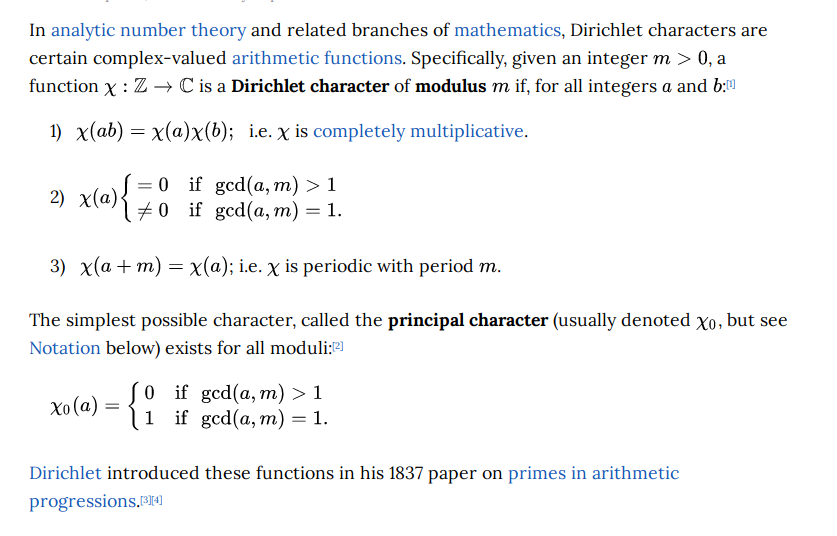• Fundamental lemma in Langlands
• Relates orbital integrals on a reductive group over a local field, to “stable” orbital integrals on its endoscopic groups.
• Endoscope: $$H\leq G$$ a quasi-split group whose Langlands dual $$H {}^{ \vee }$$ is the connected component of $$C_{G {}^{ \vee }}(x)$$ for $$x\in G {}^{ \vee }$$ some semisimple element.
• Want to get at automorphic forms and the arithmetic of Shimura varieties
• Some “stabilized” version of the Grothendieck-Lefschetz trace formula?

## 1.2 22:17

• Geometric fiber

• Reductive, semisimple, simply connected, etc for $$G\in{\mathsf{Grp}}{\mathsf{Sch}}_{/ {S}}$$: affine and smooth over $$S$$, where geometric fibers are reductive. s.s., etc algebraic groups.

• Etale morphisms

• For $$f \in \operatorname{Mor}_{\mathsf{Sch}}(X, Y)$$ finite type and $$X, Y$$ locally Noetherian, $$f$$ is etale at $$y\in Y$$ if $$f^*: {\mathcal{O}}_{f(y)} \to {\mathcal{O}}_y$$ is flat and $${\mathcal{O}}_{f(y)}/{\mathfrak{m}}_{f(y)} \to {\mathcal{O}}_{f(y)}/ f^*({\mathfrak{m}}_{f(y)} {\mathcal{O}}_y)$$ is a finite separable extension.
• Central extension

• Fiber functor

• Algebraic fundamental group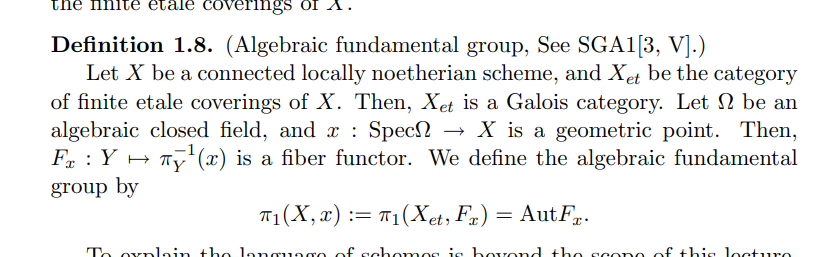• Certain groups that become isomorphic after field extensions have related automorphic representations.

• Langlands dual: $${\mathcal{L}}(G)$$ controls $${\mathsf{G}{\hbox{-}}\mathsf{Mod}}$$ somehow, arises as an extension $${ \mathsf{Gal}} (k^s _{/ {k}} ) \to {\mathcal{L}}(G) \to H$$ where $$H \in \operatorname{Lie}{\mathsf{Grp}}_{/ {{\mathbb{C}}}}$$.

• A connected reductive algebraic group over a separably closed field $$k$$ is uniquely determined by its root datum.

• Langlands dual: take root datum, dualize datum, take associated group.

• Langlands’ strategy for proving local and global conjectures: Arthur-Selberg trace formula.

• Equivalence of orbital integrals can somehow be related to Springer fibers??

• Starting point for Langlands: Artin reciprocity, generalizing quadratic reciprocity.

• Chebotarev density theorem is a generalization of Dirichlet’s theorem on arithmetic progressions.

• “The Langlands conjectures associate an automorphic representation of the adelic group $$\operatorname{GL}_n({\mathbb{A}}_{/ {{\mathbb{Q}}}} )$$ to every $$n{\hbox{-}}$$dimensional irreducible representation of the Galois group, which is a cuspidal representation if the Galois representation is irreducible, such that the Artin $$L$$-function of the Galois representation is the same as the automorphic $$L$$-function of the automorphic representation”

• Serre’s modularity conjecture: an odd, irreducible, two-dimensional Galois representation over a finite field arises from a modular form. A stronger version of this conjecture specifies the weight and level of the modular form

• $${\mathbb{A}}_{/ {{\mathbb{Q}}}}$$: keeps track of all of the completions of $${\mathbb{Q}}$$ simultaneously.

• Reciprocity conjecture: a correspondence between automorphic representations of a reductive group and homomorphisms from a Langlands group to an $$L$$-group

• Geometric Langlands: relates l-adic representations of the étale fundamental group of an algebraic curve to objects of the derived category of l-adic sheaves on the moduli stack of vector bundles over the curve.

• 2018: Lafforgue established global Langlands for automorphic forms to Galois reps for connected reductive groups over global function fields

• “Purity”: happens in a specific codimension

# 2 2021-09-12

## 2.1$${\mathsf{FI}}{\hbox{-}}$$modules (23:45)

Reference: Church-Ellenberg-Farb

• Open problem: what are the characters of representations for $$S_n$$ acting on certain vector spaces:
• $$H^*({\mathrm{Conf}}_n(X))$$
• $$H^*({ \mathcal{M}_{g} })$$ and its tautological ring $$R^*({ \mathcal{M}_{g} })$$
• Smallest subrings of Chow closed under pushforward by forgetful/gluing maps between various $${ \mathcal{M}_{g, n} }$$. Can push through the cycle class map, unknown these are isomorphic. Can get a surjection $${\mathbb{Q}}[\kappa_i] \to H^*({ \mathcal{M}_{g, n} })$$ for degree high enough.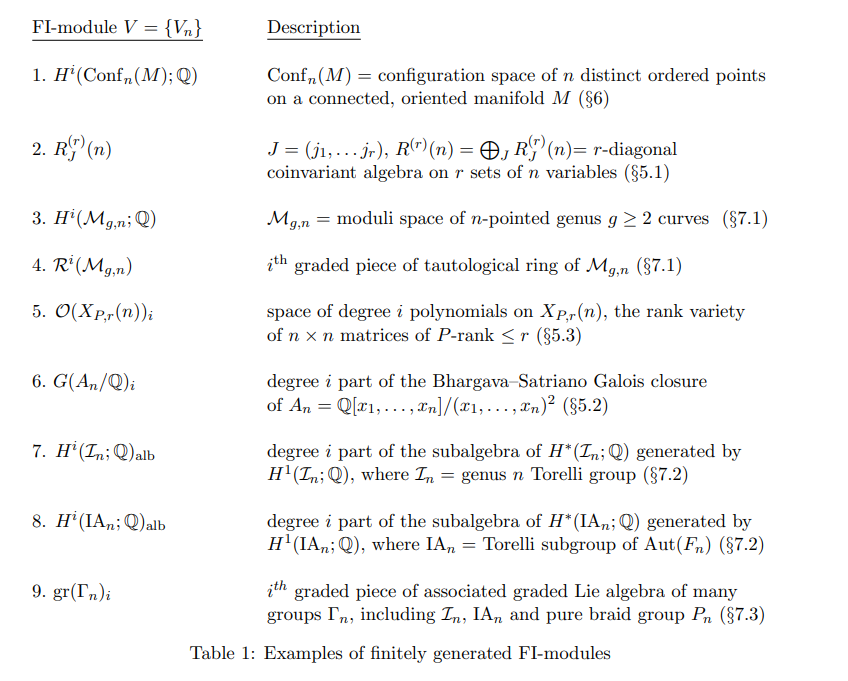• Main result: dimensions of representation stabilize.

• Sequence of $$S_n{\hbox{-}}$$reps converted into a single $${\mathsf{FI}}{\hbox{-}}$$module.

• $${\mathsf{{\mathsf{FI}}}{\hbox{-}}\mathsf{Mod}} \mathrel{\vcenter{:}}= F\in {\mathsf{Fun}}({\mathsf{FI}}, {\mathsf{k}{\hbox{-}}\mathsf{Mod}})$$.

• Any $${\mathsf{FI}}{\hbox{-}}$$module provides a linear action $$\mathop{\mathrm{End}}_{{\mathsf{FI}}}(n) = S_n$$.
• Gradings: functors from $${\mathbb{N}}\to {\mathsf{k}{\hbox{-}}\mathsf{Mod}}$$.

# 3 2021-08-05

## 3.1 Classical / Analytic Moduli Theory

• $${\operatorname{SL}}_2({\mathbb{R}})\curvearrowright{\mathbb{H}}$$ transitively by linear fractional transformations, and $${\operatorname{Stab}}(i) = {\operatorname{SO}}(2)$$. Thus one can realize $${\mathbb{H}}\cong {\operatorname{SL}}_2({\mathbb{R}})/{\operatorname{SO}}_2({\mathbb{R}})$$.

• Applying a homothety to a lattice $$\Lambda$$ yields $$L_\tau \mathrel{\vcenter{:}}={\mathbb{Z}}+ {\mathbb{Z}}\tau$$ for some $$\tau\in{\mathbb{H}}$$ and $$\Lambda \cong L_\tau$$. Writing an elliptic curve as $${\mathbb{C}}/L_\tau$$, the moduli of elliptic curves is given by \begin{align*} A_1\mathrel{\vcenter{:}}={\operatorname{SL}}_2({\mathbb{Z}})\diagdown{\mathbb{H}}\cong \dcoset{{\operatorname{SL}}_2({\mathbb{R}})}{{\operatorname{SL}}_2({\mathbb{Z}})}{{\operatorname{SO}}_2({\mathbb{R}})} .\end{align*} This quotient is Hausdorff, and $$A_1 \xrightarrow{\sim} {\mathbb{C}}$$ as topological spaces. Somehow this comes from “gluing the two bounding lines of $$F$$ and folding the circular boundary in half,” yielding the sphere minus a point.

• One can naturally compactify this by adding the point at infinity to obtain $$X(1) \mathrel{\vcenter{:}}=\overline{A_1}$$. This point is referred to as a cusp.
• $$-I$$ acts trivially on $${\mathbb{H}}$$, so this factors through $$\Gamma \mathrel{\vcenter{:}}={\operatorname{PSL}}_2({\mathbb{Z}}) \mathrel{\vcenter{:}}={\operatorname{SL}}_2({\mathbb{Z}})/\left\langle{\pm I}\right\rangle$$.

• Letting $$S= (z\mapsto -1/z) = \left(\begin{array}{cc} 0 & -1 \\ 1 & 0 \end{array}\right), T = (z\mapsto z+1) = \left(\begin{array}{ll} 1 & 1 \\ 0 & 1 \end{array}\right)$$, there are fundamental domains: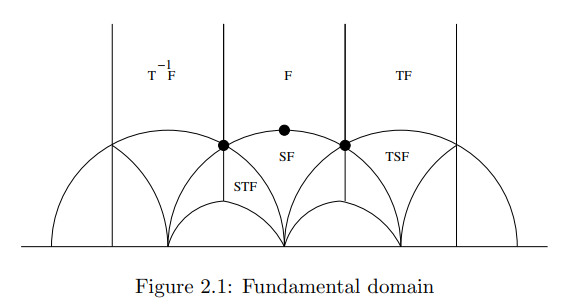• $$i$$ has isotropy $$\left\langle{S}\right\rangle$$, $$\zeta_3$$ has $$\left\langle{ST}\right\rangle$$, and $$\zeta_3^2$$ has $$\left\langle{TS}\right\rangle$$. Applying $$S$$ and $$T^{\pm 1}$$ to the fundamental domain $$F$$ tiles $${\mathbb{H}}$$ by hyperbolic triangles.

• $${\operatorname{PSL}}_2({\mathbb{Z}}) = \left\langle{S, T}\right\rangle$$.

• Maps $$f: A_1\to {\mathbb{C}}$$ are continuous iff their pullbacks along $$\pi: {\mathbb{H}}\to A^1$$ are continuous, so these are necessarily $$\Gamma{\hbox{-}}$$invariant functions.

• $$f$$ is automorphic with automorphy factor $$\phi_\gamma(z)$$ iff \begin{align*} f(\gamma z) = \phi_\gamma(z) f(z) .\end{align*} For any two such functions, their ratio $$g=f_1/f_2$$ satisfies $$g(\gamma z) = g(z)$$.

• $$f$$ is weakly modular of weight $$2k$$ if \begin{align*} f(z) = (cz+d)^{-2k} f(\gamma z), \gamma \mathrel{\vcenter{:}}={ \begin{bmatrix} {a} & {b} \\ {c} & {d} \end{bmatrix} } .\end{align*}

• Note that \begin{align*} {\frac{\partial }{\partial z}\,} {az+b \over cz +d }= {ad-bc \over (cz+d)^2} = {1\over (cz+d)^2} ,\end{align*} and so a meromorphic form $$\omega = f(z) \,dz$$ transforms under $$\gamma$$ as \begin{align*} \gamma \cdot f(z)\,dz= f(\gamma \cdot z) d(\gamma\cdot z) = (cz+d)^{-2}f(\gamma\cdot z)\,dz .\end{align*}

• So weakly modular forms of weight $$2k$$ are those form which $$\omega^{\otimes k}$$ is invariant.

• Dropping the weakly adjective involves imposing holomorphy conditions at $$\infty$$. $$f$$ is a (standard) modular form of weight $$2k$$ if $$f$$ is weakly modular, holomorphic on $${\mathbb{H}}$$, and the Fourier coefficients satisfy $$a_{<0} = 0$$.

• $$f$$ is a cusp form if $$a_0 = 0$$.
• Poisson summation: if $$f:{\mathbb{R}}\to {\mathbb{C}}$$ is a Schwartz function (smooth and super-polynomial decay), then \begin{align*} \sum_{n \in \mathbb{Z}} f(n)=\sum_{n \in \mathbb{Z}} \widehat{f}(n) .\end{align*}

• $$\zeta(s)$$ is the $$L{\hbox{-}}$$function associated to the trivial Galois representation \begin{align*} \rho_{\mathop{\mathrm{Triv}}}: G_{\mathbb{Q}}\to {\mathbb{C}}^{\times} .\end{align*} $$L{\hbox{-}}$$functions coming from arbitrary 1-dim reps will correspond to Dirichlet characters by Kronecker-Weber, and are referred to as Dirichlet $$L{\hbox{-}}$$functions.

• $$\Gamma(1) \mathrel{\vcenter{:}}={\operatorname{SL}}_2({\mathbb{Z}})$$, and principal congruence subgroups of level $$N$$ for $$\Gamma(1)$$ are defined as \begin{align*} \Gamma(N) \mathrel{\vcenter{:}}=\ker\qty{\Gamma(1) \twoheadrightarrow{\operatorname{SL}}_2({\mathbb{Z}}/N)} = \left\{{M\in \Gamma(1) {~\mathrel{\Big|}~}M\cong I \operatorname{mod}N}\right\} ,\end{align*} so the kernels of reduction mod $$N$$. Congruence subgroups are any subgroups $$H$$ such that $$\Gamma(N) \subseteq H \leq \Gamma(1)$$ for some $$N$$.

• Letting $$\Gamma(N)$$ act on $${\mathbb{H}}$$ or $${\mathbb{H}}^*$$, one can define modular curves \begin{align*} X(\Gamma) &\mathrel{\vcenter{:}}=\Gamma\diagdown {\mathbb{H}}^* \\ Y(\Gamma) &\mathrel{\vcenter{:}}=\Gamma\diagdown {\mathbb{H}} ,\end{align*} where $${\mathbb{H}}^* = {\mathbb{H}}\cup({\mathbb{Q}}\cup\left\{{\infty}\right\}) \subset {\mathbb{P}}^1({\mathbb{C}})$$.

• Note that $$Y(1)$$ parameterizes elliptic curves.
• The inclusions $$\Gamma \hookrightarrow\Gamma(1)$$ induce a branched cover $$X(\Gamma) \twoheadrightarrow X(\Gamma(1)) = A^1 \cong {\mathbb{P}}^1({\mathbb{C}})$$.

• The genera of these curves can be computed using Riemann-Hurwitz: \begin{align*} 2 g(Y)-2=(2 g(X)-2) d+\sum_{y \in Y}\left(e_{y}-1\right) ,\end{align*} yielding for $$N\geq 3$$, $$g(X(\Gamma(N)))$$ is given by \begin{align*} g=1+\frac{d(N-6)}{12 N} {\quad \operatorname{where} \quad} d=\frac{1}{2}[\Gamma(1): \Gamma(N)]=\frac{N^{3}}{2} \prod\left(1-\frac{1}{p^{2}}\right) .\end{align*}

• For $$X$$ a smooth curve and $$D\in \operatorname{Div}(X)$$, set \begin{align*} \Omega^1_X(D) \mathrel{\vcenter{:}}=\Omega^1_X \otimes{\mathcal{O}}_X(D) \cong {\mathcal{O}}_X(\omega + D) \end{align*} where $$\omega$$ is the canonical divisor. Then $${\mathsf{\Gamma}\qty{X; \Omega^1_X(D)} }$$ is the space of meromorphic 1-forms $$\omega$$ such that $$\operatorname{Div}(\omega) + D \geq 0$$ is effective.

• Define $$M_{2k}(\Gamma)$$ to be the space of weight $$2k$$ modular forms, and $$S_{2k}$$ the space of cusp forms. Then $$\bigoplus_k S_{2k} \in {\mathsf{gr}\,}^{\mathbb{Z}}{\mathsf{Alg}}_{/ {{\mathbb{C}}}} ^{\mathrm{fg}}$$, and for $${\operatorname{SL}}_2({\mathbb{Z}})$$ this algebra is generated by the Eisenstein series $$G_4$$ and $$G_6$$.

• A contravariant functor $$F$$ admits a fine moduli space $${\mathsf{B}}F$$ if $$F$$ is representable by $${\mathsf{B}}F$$, i.e. $$F({-}) \cong \operatorname{Hom}({-}, {\mathsf{B}}F)$$. By Yoneda, $$F$$ admits a universal family $${\mathsf{E}}F \to {\mathsf{B}}F$$ so that $$F(X)$$ is the pullback of it under some map $$X\to {\mathsf{B}}F$$.

• The functor $$F({-})$$ sending $$X$$ to isomorphism classes of elliptic curves over $$X$$ admits $$Y(1)$$ as a coarse moduli space and not a fine one, since there are nontrivial families with constant $$j{\hbox{-}}$$invariant. Despite this, $$E\to j(E)$$ gives a bijection between isomorphism classes of elliptic curves and points of $$Y(1)$$.

• A level $$N$$ structure is a basis for $$H_1(E; {\mathbb{Z}}/N)$$, which is symplectic since it carries a pairing with intersection matrix $${ \begin{bmatrix} {0} & {1} \\ {-1} & {0} \end{bmatrix} }$$.

• Moduli interpretations:

• $$Y_1(N) = Y(\Gamma_1(N))$$ is a coarse moduli space for pairs $$(E, P)$$ where $$P$$ is an $$N{\hbox{-}}$$torsion point.
• $$Y_0(N)$$ parameterizes $$(E, C)$$ where $$C\leq E[N]$$ is a cyclic subgroup of the $$N{\hbox{-}}$$torsion points.
• An elliptic curve $$E$$ over a scheme $$S$$ is a smooth proper map $$f:E\to S$$ with a section such that the closed fibers of $$f$$ are genus 1 curves.

• Letting $$\mathrm{Ell} (S)$$ be elliptic curves over $$S$$ up to isomorphism, $$Y(1)_{/ {{\mathbb{Z}}}} = \operatorname{Spec}{\mathbb{Z}}[j]$$ is a coarse moduli scheme for $$\mathrm{Ell} ({-})$$, and $$Y(1) = \qty{Y(1)_{/ {{\mathbb{Z}}}} { \underset{\scriptscriptstyle {\operatorname{Spec}{\mathbb{Z}}} }{\times} } \operatorname{Spec}{\mathbb{C}}}^{\mathrm{an}}$$ is the associated analytic space.
• Look up the \begin{align*}\[Weil Pairing\end{align*} ] $$e_n$$.

• A level $$N$$ structure is a pair of points $$P, Q \in E[N]$$ generating a subgroup with that $$e_n(P, Q) = \zeta_N$$ is a primitive $$N$$th root of unity. More generally, for curves over schemes, this is a pair of sections inducing level structures on closed fibers.

• For $$N=2$$, $$Y(2) = \operatorname{Spec}{\mathbb{Z}} { \left[ {t, {1\over t(t-1)}} \right] }$$ as a coarse moduli space, and a corresponding almost-universal family $$y^2z = x(x-z)(x-tz)$$.

• For $$N=3$$, $$Y(2) = \operatorname{Spec}R { \left[ {t, {1\over t^3-1}} \right] }$$ where $$R \mathrel{\vcenter{:}}={\mathbb{Z}} { \left[ {{1\over 3}, \zeta_3^2} \right] }$$ for $$\zeta_3$$ a primitive third root of unity. The universal family is $$x^3 + y^3 + z^3 = 3txyz$$, where the level 3 structure is given by the sections $${\left[ {-1, 0, 1} \right]}, [-1, \zeta_3^2, 0]$$.

## 3.2 Moduli as Stacks

• Can view an elliptic curve as a pair $$(X, p)$$ where $$X$$ is a compact Riemann surface with $$\dim_{\mathbb{C}}H^0(X; \Omega^1_X) = 1$$ and $$p$$ is a point.

• Why elliptic curves have 1-dimensional homology: any globally defined holomorphic 1-form is a double periodic holomorphic 1-form on $${\mathbb{H}}$$, forcing it to be constant by Liouville. :
• Can define a lattice $$\Lambda \subseteq V$$ in an arbitrary vector space as a discrete cocompact subgroup, so $$V/\Lambda$$ is compact.

• The order of a function $$f$$ at $$x$$ is given by

\begin{align*} \text { ord }_{x}(f):= \begin{cases}0 & \text { if } \mathrm{f} \text { is holomorphic and non-zero at } x \\ k & \text { if } \mathrm{f} \text { has a zero of order } k \text { at } x \\ -k & \text { if } \mathrm{f} \text { has a pole of order } k \text { at } x\end{cases} .\end{align*}

• Can define divisors as maps $$D:X\to {\mathbb{Z}}$$ where cofinitely many points are sent to zero. The map $${\operatorname{Ord}}_{{-}}(f)$$ is a divisor associated to any function $$f$$, denoted $$(f)$$.

• Divisors $$(f)$$ for $$f$$ meromorphic are principal, and setting $$\deg(\sum n_i p_i) \mathrel{\vcenter{:}}=\sum n_o$$, it turns out that $$\deg((f)) = 0$$ for $$f$$ principal.

• As a consequence, meromorphic functions have equal numbers of zeros and poles, and 1-forms that are not identically zero can not have zeros.
• The period map is defined as \begin{align*} \Phi: H_{1}(X, \mathbb{Z}) & \rightarrow \mathbb{C} \\ \gamma & \mapsto \int_{\gamma} \omega .\end{align*} For a fixed nonzero holomorphic 1-form $$\omega$$, there is a group pf periods which forms a lattice over $${\mathbb{C}}$$: \begin{align*} \Lambda \mathrel{\vcenter{:}}=\left\{{\int_\gamma \omega \in {\mathbb{C}}{~\mathrel{\Big|}~}\gamma \in H_1(X; {\mathbb{Z}})}\right\} = \operatorname{im}\Phi .\end{align*} One can recover $$(X, P)$$ as $$({\mathbb{C}}/\Lambda(\omega), 0)$$

# 5 Fargues-Fontaine Stuff

Useful references:

## 5.1 Motivation / Summary

Some notes on the Fargues-Fontaine curve $${X_\mathrm{FF}}$$, the fundamental curve of $$p{\hbox{-}}$$adic Hodge theory.

What’s the point? There’s supposed to be a “curve” $${X_\mathrm{FF}}$$ over $${ {\mathbb{Q}}_p }$$ where local Langlands for $${ {\mathbb{Q}}_p }$$ should be encoded as geometric Langlands on $${X_\mathrm{FF}}$$, which glues together important period rings from $$p{\hbox{-}}$$adic Hodge theory. Stems from conjectures of Grothendieck wanting to related de Rham cohomology to étale cohomology, and a similar theorem proved by Faltings in the 80s. Hot topic right now because of a conjecture related to local Langlands: supposed to give a way to go from the Galois side to the automorphic side. Important object: $${\mathsf{Bun}}_G$$, the moduli stack of $$G{\hbox{-}}$$bundles for $$G$$ a reductive algebraic group over a local field over a family of $${X_\mathrm{FF}}$$ curves.

A useful overall analogy: it’s like the Riemann sphere $${\mathbb{CP}}^1 \mathrel{\vcenter{:}}={\mathbb{P}}^1({\mathbb{C}})$$, and in fact the adic version is a $$p{\hbox{-}}$$adic Riemann surface. The full ring of meromorphic functions on $${\mathbb{P}}^1({\mathbb{C}})$$ is $${\mathbb{C}}\qty{z}$$, but $${\mathbb{C}}[z]$$ captures most of the data away from $$\infty$$. $${\mathbb{C}}[z]$$ as a $${\mathbb{C}}{\hbox{-}}$$algebra consists of regular (polynomial) functions on $${\mathbb{P}}^1({\mathbb{C}})$$ with a pole at $$\infty$$ of order equal to the degree of the polynomial. View the $${\mathbb{Z}}{\hbox{-}}$$algebra $${\mathbb{Z}}$$ as the regular functions on $$P$$ the set of primes (finite places), with a point at $$\infty$$ (infinite place) given by the usual valuation $${\left\lvert {{-}} \right\rvert}$$. Make this more algebro-geometric by replacing $${\mathbb{Z}}$$ with either $${ {\mathbb{Z}}_p }$$ or $${ {\mathbb{Q}}_p }$$ (so $$p{\hbox{-}}$$adic things) and looking at $${ {\mathbb{Q}}_p }{\hbox{-}}$$algebras $$B$$ as replacements for regular functions. $${X_\mathrm{FF}}$$ is also supposed to “geometrize” period rings from $$p{\hbox{-}}$$adic Hodge theory. One can also geometrize class field theory somehow, and realize $${ \mathsf{Gal}} (\mkern 1.5mu\overline{\mkern-1.5mu{\mathbb{Q}}\mkern-1.5mu}\mkern 1.5mu/{\mathbb{Q}})$$ as some kind of fundamental group.

$${X_\mathrm{FF}}$$ is also roughly a moduli of “untilts,” which allow passing between $${\mathbb{F}}_p$$ and $${ {\mathbb{Q}}_p }$$. A major goal is to go from characteristic zero to characteristic $$p$$ (relatively easy) and then to go back to characteristic zero (relatively hard). The curve is useful because many linear algebraic objects of $$p{\hbox{-}}$$adic theory can be translated into vector bundles over $${X_\mathrm{FF}}$$, and there is “Grothendieck splitting” type of theorem for those.

Todos: motivate

• Perfectoid spaces
• Tilts/untilts
• Galois representations (might have notes elsewhere..?)
• Langlands, period!
• Absolute Galois groups, particularly $$G({ \mkern 1.5mu\overline{\mkern-1.5mu \mathbb{Q} \mkern-1.5mu}\mkern 1.5mu }/{\mathbb{Q}})$$ (see notes elsewhere)
• Relations to homotopy theory: an isomorphism of Lubin-Tate, and the Drinfeld tower?

### 5.1.1 Definitions

• Curve: over $$k= { \mkern 1.5mu\overline{\mkern-1.5muk\mkern-1.5mu}\mkern 1.5mu }$$, an integral separated scheme of finite type over $$k$$ of dimension 1.

• Finite type: on affines, when $$\operatorname{Spec}A\to \operatorname{Spec}B$$ induces $$B\to A$$ making $$A \in \mathsf{B}{\hbox{-}}\mathsf{Mod}$$ finitely generated.

• Algebraic curve: $$X$$ of pure Krull dimension 1, or equivalently $$X$$ has an affine open cover $$\left\{{\operatorname{Spec}R_i}\right\}_{i\in I} \rightrightarrows X$$ where each $$\operatorname{Spec}R_i$$ is Krull dimension 1.

• For (irreducible) varieties over $$k= { \mkern 1.5mu\overline{\mkern-1.5muk\mkern-1.5mu}\mkern 1.5mu }$$, just an algebraic variety of dimension 1.
• Complete curve: for algebraic varieties $$X$$, universally closed: the projections $$X\times({-})\to({-})$$ are closed maps when evaluated on any variety.

Equivalently, $$X\to k$$ is a proper morphism (separated, finite type, and universally closed)

• For topological spaces, $$X$$ is compact iff $$X$$ is complete.
• Complete (smooth) varieties of dimension 1 are always projective.
• Completely valued field: a field $$k$$ equipped with a valuation $$v$$, where $$k$$ is complete as a metric space with respect to $$v$$.

• Valuation: a group morphism $$(K, \cdot) \to ({\mathbb{R}}_{\geq 0}, +)$$ with $$v(a) = \infty \iff a =0$$ satisfying an ultrametric triangle inequality.

• Almost a metric. Supposed to capture the multiplicity of zeros/poles of a function.
• Valuation ring: defined as $${\mathcal{O}}_K \mathrel{\vcenter{:}}=\left\{{x\in K {~\mathrel{\Big|}~}v(x) \leq 1}\right\}$$, the unit disc with respect to the valuation.

• Has a unique maximal ideal, the interior of the disc: $${\mathfrak{m}}\mathrel{\vcenter{:}}={\mathcal{O}}_K\setminus\left\{{v(x) = 1}\right\}$$.
• Dedekind scheme: a Noetherian integral scheme of dimension 1 where every local ring is regular.

• Example: $$\operatorname{Spec}R$$ for $$R$$ a Dedekind domain.
• Slogan: any non-generic point is a closed point.
• Tilts: for $$k$$ a field, denoted \begin{align*} k^ { \flat } \mathrel{\vcenter{:}}=\varprojlim(k\xrightarrow{x\mapsto x^p} k \xrightarrow{x\mapsto x^p} k \cdots) ,\end{align*} realized as certain sequences $$\left\{{x_n}\right\}$$ where $$x_{n+1}^p = x_n$$, made into a characteristic $$p$$ field with pointwise multiplication and a $$p{\hbox{-}}$$twisted addition law involving limits.

• Idea: an inverse limit of applying Frobenius. Yields a perfect $${\mathbb{F}}_p{\hbox{-}}$$algebra. Equipped with a valuation and a valuation ring.
• Untilts: For $$k$$ a field, a pair $$(K, \iota)$$ where $$\iota: k\xrightarrow{\sim} K^ { \flat }$$ is an isomorphism of fields, plus a condition on valuation rings $${\mathcal{O}}_k$$ and $${\mathcal{O}}_K^ { \flat }$$.

• Formal schemes: topologically ringed spaces $$(X, {\mathcal{O}}_X)$$ where $${\mathcal{O}}_X$$ is a sheaf of topological rings, which is locally a formal spectrum of a Noetherian ring.

• Formal spectrum: $$\operatorname{Spf}R \mathrel{\vcenter{:}}=\operatorname{Spf}_I R = \colim_{n} \operatorname{Spec}R/I^n$$, where $$I^n$$ is a system of ideals forming neighborhoods of zero in the sense that if $$U\ni 0$$ then $$I^n \subseteq U$$ for some $$n$$. Take the colimit in $${\mathsf{Top}}\mathsf{RingSp}$$. The structure sheaf of $$\operatorname{Spf}_I R$$ is $$\colim_{n} {\mathcal{O}}_{\operatorname{Spec}R/I^n}$$

• Meant to accommodate formal power series as regular functions.
• Examples: $$I{~\trianglelefteq~}R$$, take the $$I{\hbox{-}}$$adic topology with basic open sets $$r + I^n$$. Then $${ {\left\lvert {\operatorname{Spf}A} \right\rvert} } = { {\left\lvert {\operatorname{Spec}A/I} \right\rvert} }$$, so the underlying point-set spaces are the same.
• Formal disk: an infinitesimal thickening of a point.

• Punctured formal disk: remove the unique global point from a formal disk.

• adic space: for $$X$$ a variety over a nonarchimedean field, e.g. $${ {\mathbb{Q}}_p }$$, the associated analytic space $$X^{\mathrm{an}}$$.

• Periods: the results of integrating an algebraic differential form in $$H_\mathrm{dR}^*$$ over a cycle in singular cohomology $$H_{{\mathrm{sing}}}^*$$. Just a number in $${\mathbb{C}}$$!

• Witt vectors: complicated construction, similar to $$p{\hbox{-}}$$adic integers. Uniquely characterized as a lift $$W(K)$$ of a perfect $${\mathbb{F}}_p{\hbox{-}}$$algebra $$K$$ to $${ {\mathbb{Z}}_p }{\hbox{-}}$$algebra, which becomes $$p{\hbox{-}}$$adically complete and $$p{\hbox{-}}$$torsionfree. Also lifts Frobenius.

• Perfectoid spaces: ? Frobenius is an isomorphism? Plus other conditions?

• For rings, there is a natural map in $$p{\hbox{-}}$$adic Hodge theory $${\mathbb{A}}_\inf(S) \to S$$, and if $$S$$ is perfectoid this is supposed to look like a 1-parameter deformation (“pro-infinitesimal”).
• If $$\char S = p$$, then $$S$$ is perfectoid iff $$S$$ is perfect, i.e. Frobenius is an automorphism.
• If $$K$$ is a perfectoid field, then $$G(\mkern 1.5mu\overline{\mkern-1.5muK\mkern-1.5mu}\mkern 1.5mu/K) \cong G(\mkern 1.5mu\overline{\mkern-1.5muK^ { \flat } \mkern-1.5mu}\mkern 1.5mu/K^ { \flat } )$$, so we can study absolute Galois groups here (result of “almost purity” theorems).
• Mixed characteristic: a ring $$R$$ with an ideal $$I{~\trianglelefteq~}R$$ with $$\char R = 0$$ but $$\char R/I = p > 0$$. The motivating examples: $${\mathbb{Z}}$$, or $${\mathcal{O}}_K$$ for $$K$$ a number field, $${ {\mathbb{Z}}_p }$$.

### 5.1.2 Main Results

• Why care about tilts/untilts: for $$k$$ a field and $$X\mathrel{\vcenter{:}}=\operatorname{Spec}{\mathbb{Q}}$$ as a scheme, characteristic zero tilts of $$k$$ are a good replacement for $$X(k)$$ which makes $$X\times X$$ nontrivial.

• Main theorem: if $$k = { \mkern 1.5mu\overline{\mkern-1.5muk\mkern-1.5mu}\mkern 1.5mu }$$ is a completely valued characteristic $$p$$ field, then there exists a Dedekind scheme $$X \to \operatorname{Spec}{ {\mathbb{Q}}_p }$$ whose closed points $$x$$ correspond to (isomorphism classes) of characteristic 0 untilts of $$k$$, modulo the action of the Frobenius $$\varphi(x) = x^p$$.

• So moduli of untilts of a perfectoid field, behaves like open disc in $${\mathbb{C}}$$. The unique characteristic $$p$$ untilt is like $$z=0 \in {\mathbb{C}}$$.
• Why the FF “curve” isn’t a curve: it’s a scheme over $${ {\mathbb{Q}}_p }$$, but not finite-type: specifically, the structure morphism $$X\to \operatorname{Spec}{ {\mathbb{Q}}_p }$$ is not finite type.

• Why not finite type? Since if $$x\in X$$ is a closed point then the residue field $$\kappa(x) / { {\mathbb{Q}}_p }$$ is not a finite extension.
• Incidentally, $$\kappa(x)$$ is exactly the untilt of $$k$$ corresponding to $$x$$.
• Nice properties of $${X_\mathrm{FF}}$$ coinciding with curves:

• $$\sum \deg_f(x) = 0$$ for every rational function $$f$$ on $${X_\mathrm{FF}}$$, similar to being projective/complete as a scheme/variety.
• Note: very similar to a theorem from complex analysis: sum of orders of poles and zeros equals zero for a meromorphic function on $${\mathbb{P}}^1({\mathbb{C}})$$.
• Line bundles are classified by degree
• $$H^1(C, {\mathcal{O}}_C) = 0$$, similar to having genus $$g=0$$.
• Usually it’s like $$2g = \operatorname{rank}H^1$$
• The structure morphism $$X\to \operatorname{Spec}{ {\mathbb{Q}}_p }$$ has simply connected fibers
• Useful heuristics: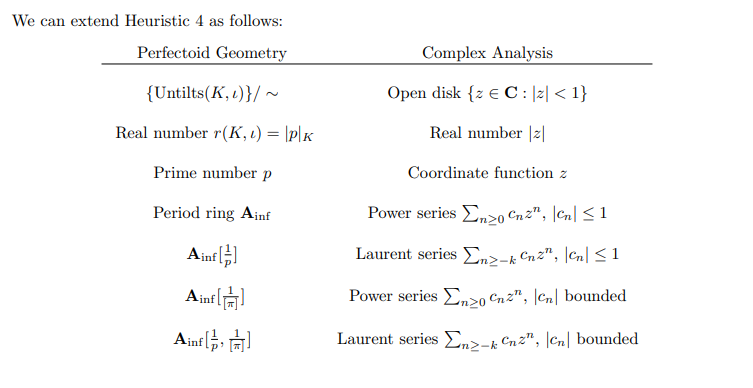## 5.2 Constructing the curve

Notation:

• $$B_{\varepsilon}\mathrel{\vcenter{:}}= B^{\varphi = 1}_{\mathrm{crys}}$$
• $${\mathbb{A}}_\inf(K) \mathrel{\vcenter{:}}= W(K^ { \flat } )$$, i.e. just Witt vectors of the tilt.
• Supposed to interpolate between the $$\char 0$$ geometry of $$K$$ and $$\char p$$ geometry of $$K^ { \flat }$$.
• Importantly, even if $$K$$ doesn’t have Frobenius, $$K^ { \flat }$$ does! This will yield an action of Frobenius on cohomology – this is probably used to set up trace formulas.

### 5.2.1 Method 1: Schematically, Proj Construction

• Punchline: $${X_\mathrm{FF}}= \mathop{\mathrm{Proj}}\qty{\bigoplus_{n\geq 0} B^{\varphi = p^n}}$$ as a scheme.

• $$B^{\varphi = p^n}$$ are the elements in $$B$$ where $$\varphi(x) = p^n x$$ where $$\varphi$$ is the Frobenius.

• $$B \mathrel{\vcenter{:}}=\colim_{I\subseteq [0, 1] \subset {\mathbb{R}}} B_{I}$$ is a $$p{\hbox{-}}$$adic Frechet space

• Supposed to look like holomorphic functions of $$p$$.
• For $$I\mathrel{\vcenter{:}}=[a, b]$$, $$B_{[a, b]}$$ is the completion of $$W({\mathcal{O}}_C^ { \flat } ) { \left[ {{1\over p}, {1\over {\left\lvert {\pi } \right\rvert}}} \right] }$$ with respect to Gauss norms, where $$W$$ denotes the Witt vectors, and we’ve localized at $$p$$ and $$\pi$$ a pseudo-uniformizer.

• Other notation used:

• Alternatively using the Riemann sphere analogy: $${\mathbb{P}}^1({\mathbb{C}})$$ can be recovered as a proj. Write $${\operatorname{Fil}}_k {\mathbb{C}}[z] \mathrel{\vcenter{:}}=\left\{{f\in {\mathbb{C}}[z] {~\mathrel{\Big|}~}\deg f \leq k}\right\}$$ to be the $$k$$th filtered piece using a filtration by degree.

• The claim is that $${\mathbb{P}}_1({\mathbb{C}}) \cong \mathop{\mathrm{Proj}}\qty{\bigoplus_{k\geq 0} {\operatorname{Fil}}_k {\mathbb{C}}[z]}$$.

• Somehow this uses that $${\mathbb{C}}[z_0, z_1] \xrightarrow{\sim} \bigoplus_{k\geq 0} {\operatorname{Fil}}_k {\mathbb{C}}[z]$$, no clue..
• By analogy, maybe $${X_\mathrm{FF}}= \mathop{\mathrm{Proj}}\qty{ \bigoplus_{k\geq 0} {\operatorname{Fil}}_k B_{\varepsilon}}$$ where $${\operatorname{Fil}}_k B_{\varepsilon}\mathrel{\vcenter{:}}=\left\{{b\in B_{\varepsilon}{~\mathrel{\Big|}~}v(b) \geq k}\right\}$$, where we take a valuation $$v$$ coming from $$B_\mathrm{dR}$$.

• There is a subalgebra $$B_{\mathrm{crys}}^+ \subseteq B_{\mathrm{crys}}$$ where $$B_{\mathrm{crys}}^+{ \left[ { \frac{1}{t} } \right] } \cong B_{{\mathrm{crys}}}$$ for some $$t$$, plus some other properties.

• Can then obtain \begin{align*} {X_\mathrm{FF}}= \mathop{\mathrm{Proj}}\qty{\bigoplus _{k\geq 0} \qty{B_{\mathrm{crys}}^+}^{\varphi = p^k}} .\end{align*}

#### 5.2.1.1 Informal Description

Glue together period rings: an affine scheme to a formal disk along a formal punctured disc, so like \begin{align*} {X_\mathrm{FF}}= \operatorname{Spec}B_{{\mathrm{crys}}}^{\varphi = 1} { \displaystyle\coprod_{\operatorname{Spec}B_{\mathrm{dR}}} } \operatorname{Spec}B_{\mathrm{dR}}^+ .\end{align*}

• $$\operatorname{Spec}B_{{\mathrm{crys}}}^{\varphi = 1}$$ is an affine scheme.

• $$\operatorname{Spec}B_{\mathrm{dR}^+}$$ is a formal disk.

• $$\operatorname{Spec}B_{\mathrm{dR}}$$ is a formal punctured disk.

• $$B_\mathrm{dR}$$ is a period ring, cooked up to relate $$H^*_\mathrm{dR}(Y)$$ to $$H^*_{\text{ét}}(Y_{\mkern 1.5mu\overline{\mkern-1.5muK\mkern-1.5mu}\mkern 1.5mu}; { {\mathbb{Q}}_p })$$ when $$Y$$ is the generic fiber of a scheme over $$W(k)$$ and $$Y_{\mkern 1.5mu\overline{\mkern-1.5muK\mkern-1.5mu}\mkern 1.5mu} \mathrel{\vcenter{:}}= Y { \underset{\scriptscriptstyle {\operatorname{Spec}K} }{\times} } \operatorname{Spec}\mkern 1.5mu\overline{\mkern-1.5muK\mkern-1.5mu}\mkern 1.5mu$$ (see Crystalline comparison conjecture).

• $$B_{\mathrm{dR}}$$ is a complete DVR, a field, and a (very large) $${ {\mathbb{Q}}_p }{\hbox{-}}$$algebra with residue characteristic zero, and $$B_{{\mathrm{crys}}} \leq B_{\mathrm{dR}}$$ is a subring.
• $$B_\mathrm{dR}^+$$ is its valuation ring (or place), i.e. a subring such that for every element $$x$$ of $$\operatorname{ff}(B_{\mathrm{dR}})$$, either $$x \in B_{\mathrm{dR}}^+$$ or $$x^{-1}\in B_{\mathrm{dR}}^+$$.

• This is supposed to be like a ring of integers $${\mathcal{O}}_K$$ for a number field $$K$$. It is non-canonically isomorphic to the $$p{\hbox{-}}$$adic Laurent field $${\mathbb{C}}_p((t))$$.
• So it’s like the one-point compactification of $$\operatorname{Spec}$$ of the period ring of $$p{\hbox{-}}$$adic Hodge theory.

• This is supposed to look like building the Riemann sphere $${\mathbb{P}}^1({\mathbb{C}})$$ as $${\mathbb{C}}[z]$$ (regular away from $$\infty)$$ glued to $${\mathbb{C}}\dqty{1\over z}$$ (germs of meromorphic functions at $$\infty$$).

• $$B_{{\mathrm{crys}}}^{\varphi = 1}$$ is supposed to look like $${\mathbb{C}}[z]$$. Classically, $${\mathbb{C}}\qty{z}$$ is the full field of meromorphic functions on $${\mathbb{CP}}^1$$, and $${\mathbb{C}} { \left[ {z} \right] }$$ are regular functions on $${\mathbb{CP}}^1\setminus\left\{{\infty}\right\}$$.
• And $$B_\mathrm{dR}$$ is like $${\mathbb{C}}\dqty{1\over z}$$, germs of meromorphic functions at infinity.
• Taking $${\mathbb{CP}}^1$$ and puncturing at a point yields a disc, so $${\mathbb{CP}}^1\setminus\left\{{\infty}\right\}, {\mathbb{CP}}^1\setminus\left\{{0}\right\}$$ are discs. Their overlap is $${\mathbb{CP}}^1\setminus\left\{{\infty, 0}\right\} \cong {\mathbb{D}}\setminus\left\{{0}\right\}$$, a disc punctured at $$z=0$$.